# [京哥读论文]之From Hashing to CNNs: Training Binary Weight Networks via Hashing

## 主要贡献

• 本文揭示了保持内积哈希与BWN之间的紧密关联。
• 为了减轻用哈希方法所带来的loss，本文将binary codes乘以了一个scaling factor并用交替优化的策略来更新binary codes以及factor。
• 在Cifar10,Cifar100以及ImageNet上实验，本文提出的BWNH方法比之前方法要好。

## Inner-product preserving hashing

$min\quad \Vert S-h(X)^Tg(W)\Vert^2_F \quad (1)$
$h(\cdot)$$g(\cdot)$表示的是向量$X$$W$的哈希函数。

## 哈希与BWN之间的关联

$min \quad L(B)=\Vert W-B\Vert^2_F \quad s.t.\;B\in\{+1,-1\}^{S\times N} \quad (2)$

$min\quad L(B)=\Vert X^TW-X^TB\Vert^2_F \quad s.t. \; B\in\{+1,-1\}^{S\times N} \quad (3)$

$min\quad L(A,B)=\Vert S-X^TBA\Vert_F^2=\sum_i^N\Vert S_i-\alpha_i\cdot X^TB_i\Vert_F^2\quad (5)$
$S=X^TW, S_i\in \mathbb R^{M\times 1}$是向量$S$的第$i$列向量。等式$(5)$又可以分为N个独立子问题:
$min\quad L_i(a_i,B_i)=\Vert S_i-\alpha_i \cdot X^TB_i\Vert_F^2\quad s.t.\; B_i\in\{+1,-1\}^{S\times 1}\quad (6)$

$min L_i(\alpha_i)=const+\alpha_i^2\Vert X^TB_i\Vert_F^2-2\alpha_iS_i^TX^TB_i \quad (7)$
$\alpha_i$进行求导，$\partial L_i (\alpha_i) \over \partial \alpha_i KaTeX parse error: Can't use function '$' in math mode at position 54: …X^TB_i\quad (8)$̲令其等于0，则$\alpha_…\alpha_i={S_iTXTB_i \over \Vert XTB_i\Vert_F2}\quad (9)KaTeX parse error: Can't use function '$' in math mode at position 8: **最后更新$̲B_i固定\alpha_i$*…min\quad L_i(B_i)=const+\Vert ZTB_i\Vert_F2-2Tr(B_i^Tq) \quad s.t.; B_i\in{+1,-1}^{S\times 1}\quad (10)KaTeX parse error: Can't use function '$' in math mode at position 5: 在这里$̲Z=\alpha \cdot …min\quad (B_i’^TZ’v-q_j)b\quad s.t.; b\in{+1,-1} \quad (11)KaTeX parse error: Can't use function '$' in math mode at position 10: 这样我们可以得到$̲B_i$的第$j$个元素的解：…b=sign(q_j-B_i’^TZ’v)\quad (12)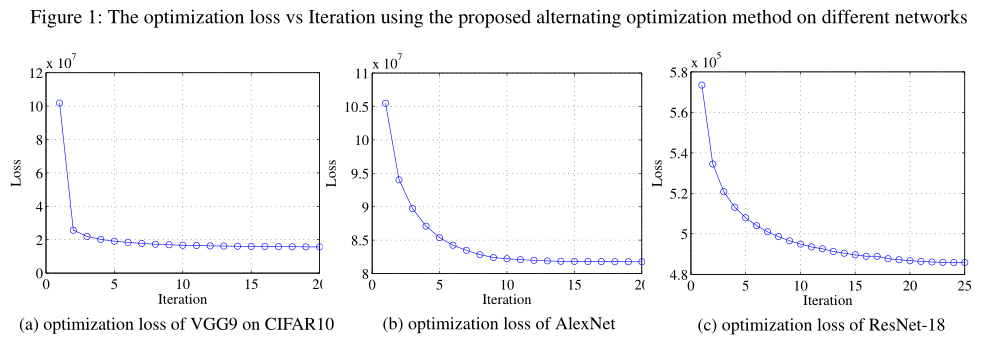## Layer-wise optimization

$min\;L(A,B)=\Vert(X^{l+1})^TW^{l+1}-(\widetilde{X}^{l+1})^TB^{l+1}A^{l+1}\Vert^2_F=\Vert S^{l+1}-(\widetilde{X}^{l+1})^TB^{l+1}A^{l+1}\Vert_F^2\quad (13)$

## The While Training Scheme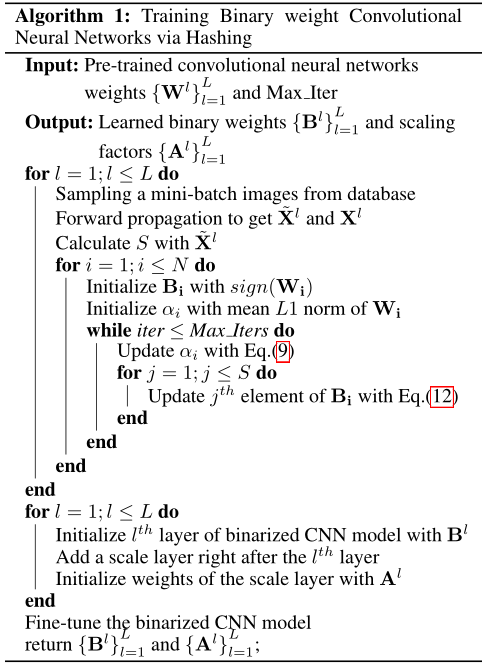## Experiments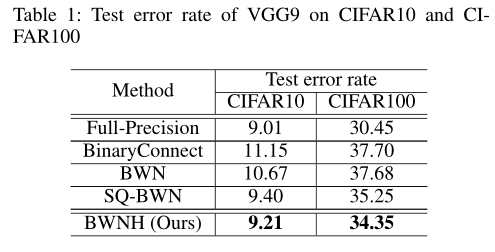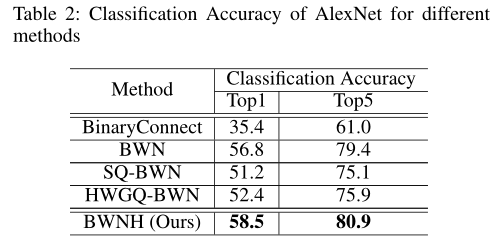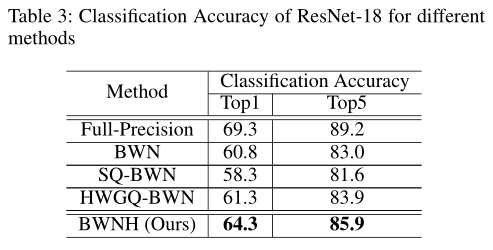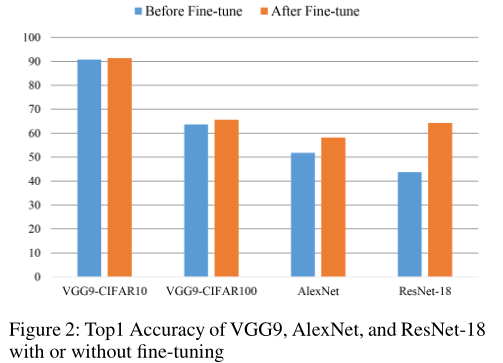##The Effect of Scaling Factor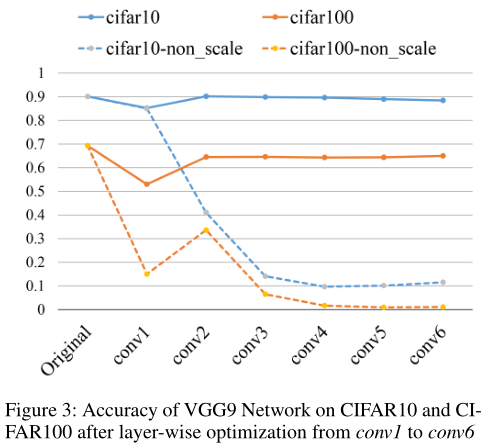©️2019 CSDN 皮肤主题: 技术黑板 设计师: CSDN官方博客